# Poly Decomp

## Mark Bayazit's Algorithm

### Evolution

My algorithm was built incrementally based on a few simple observations:

• A polygon can be broken into convex regions by eliminating all reflex vertices
• Eliminating two reflex vertices with one diagonal is better than eliminating just one
• A reflex vertex can only be removed if the diagonal connecting to it is within the range given by extending its neighbouring edges; otherwise, its angle is only reduced

From this, the algorithm naturally presents itself. Start at an arbitrary vertex, and iterate around the polygon until we find a reflex vertex i. Extend the edges incident at i until they hit an edge, like so: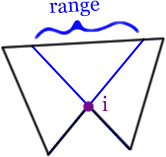#### Case 1

If there are no vertices in this range, create a new (Steiner) vertex in the center and connect to that.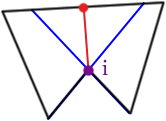#### Case 2

If there are one or more vertices in this range, connect vertex i to the closest one.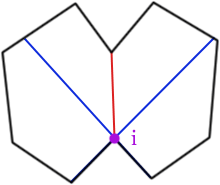#### Case 3

However, sometimes this can fail.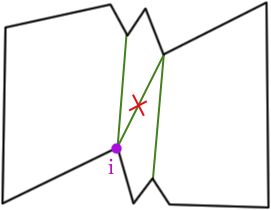This can easily be fixed with a post-processing or cleanup step by iterating over all the added diagonals and removing the ones that are no longer needed.

#### Case 4

As pointed out by Dr. Bhattacharya, this does not always work either.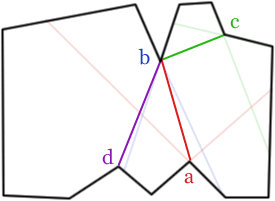Edges ab and bc could be replaced with ac for a better solution. This can be fixed too, however. Notice that vertex b is in c's range, but c is not in b's range. However, a is in c's range and vice versa; this means that both reflex vertices can be removed with a single diagonal. Therefore, the diagonal ac should be preferred over the diagonal bc.

Thus, vertex i should connect to the vertex with the highest priority. From highest to lowest the priorities are:

• closest reflex vertex that can also be eliminated (it's better to eliminate two than one)
• closest reflex vertex (it's better to reduce the angle of a reflex than not at all -- this will give us more choices when trying to eliminate it later)
• closest vertex (less chance of the new diagonal intersecting an existing edge)

#### Case 5

The reason for using the closest vertex was that I thought it couldn't be blocked. However, I overlooked this case: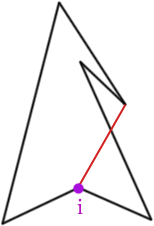Again, this can easily be fixed with a visibility check. It does make the algorithm more complex, however.

### Shortcomings

With all these checks in place, my algorithm should produce the optimal solution most of the time and is still asymptotically faster than existing solutions. However, there are still two general cases where it will fail, and are not easily resolvable.

#### Case 1

My algorithm is not optimal for all solutions that require Steiner points. For example, it cannot handle four leaf clover-like polygons.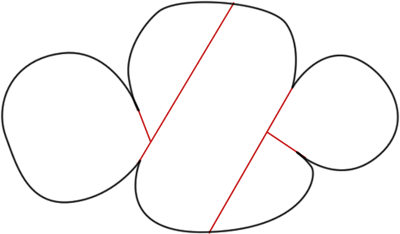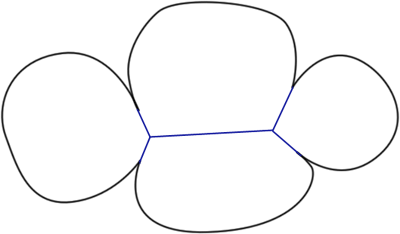#### Case 2

Worse yet, the "closest vertex" heuristic can be exploited to make my algorithm produce poor results for cases without Steiner points too.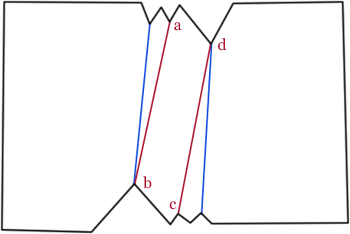Diagonals ab and cd, could be replaced with a single diagonal ac to reduce the solution by one polygon.

### Optimality

This algorithm uses Steiner points, but is not optimal for either case (with or without Steiner points). However, it does tend to produce optimal results most of the time, in practice.

### Complexity

• It takes O(n) time to find all r reflex vertices.
• It takes 2*O(n) time to extend the incident edges of a reflex vertex (the time to check a ray against all edges)
• It can take up to O(n) time to check all the vertices in range for the best candidate
• The algorithm is recursive, but since it eliminates one reflex vertex during each iteration, and only takes O(n) time per reflex vertex, the entire algorithm takes O(nr) time.

### Improvements

One way to get around the last case is to form a hybrid method with Keil's algorithm. Vertex a could have been connected to vertices b, c, or e and have made them both non-reflex in each case. Thus, we could try all three of them, and take the best solution, just as in Keil's algorithm. If speed is a concern, we could only examine only, say, the top five highest priority vertices.

### Screenshots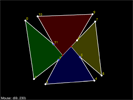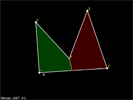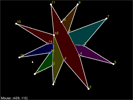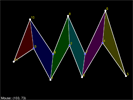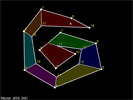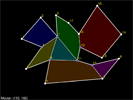polydecomp-bayazit.zip (268 kB)

Tested on Ubuntu 8.10 64-bit.

Requirements:
Libraries: FTGL (for fonts), FreeType2 (needed by FTGL), GLFW (for windows and keyboard input), OpenGL
Software: CMake (if it doesn't build)

To build and run:

```cmake . make ./conv```

Update Oct 2015: Source code can now be found on BitBucket.